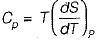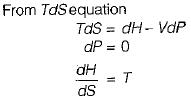Courses

# Thermodynamic Relations - 2

## 10 Questions MCQ Test Topicwise Question Bank for GATE Mechanical Engineering | Thermodynamic Relations - 2

Description
This mock test of Thermodynamic Relations - 2 for Mechanical Engineering helps you for every Mechanical Engineering entrance exam. This contains 10 Multiple Choice Questions for Mechanical Engineering Thermodynamic Relations - 2 (mcq) to study with solutions a complete question bank. The solved questions answers in this Thermodynamic Relations - 2 quiz give you a good mix of easy questions and tough questions. Mechanical Engineering students definitely take this Thermodynamic Relations - 2 exercise for a better result in the exam. You can find other Thermodynamic Relations - 2 extra questions, long questions & short questions for Mechanical Engineering on EduRev as well by searching above.
QUESTION: 1

### Joule Thomson coefficient (μ) is given by

Solution:

Joule Thomson coefficientQUESTION: 2

### Gibb's free energy (G) is given by

Solution:

Gibb’s free energy (G ) = H - TS

QUESTION: 3

### Helmholtz function (F) is given by

Solution:

Helmholtz function F = U - TS

QUESTION: 4

Which one of the following expression is true for Tds equal to

Solution:

Tds = du+ PdV— First Tds equation .
Tds - dh- VdP— Second Tds eauation

QUESTION: 5

Gibb’s free-energy functions is a property comprises

Solution:

Gibbs free energy function (G)
G = H - TS = f(H, T, S)

QUESTION: 6

The specific heat at constant pressure (Cp) is given by

Solution:

From TdS equation
TdS = dh - VdP
for constant pressure process
dP= 0
Since dh = cpdT
TdS = CpdTQUESTION: 7

For real gases Cp = Cv at

Solution:
QUESTION: 8

The Ciausius-clapeyron equation gives the slope of a curve in

Solution:
QUESTION: 9

Rate of change of enthalpy with respect to entropy at constant pressure for a perfect gas

Solution:QUESTION: 10

The number of components (C), Phases (P) and degree of freedom (F) are related by Gibb’s phase rule as

Solution:

Gibb’s phase rule is given b
C + 2 = P + F
P + F - C - 2 = 0